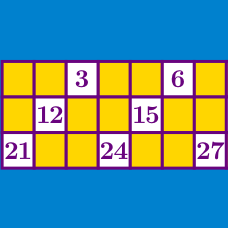Number Theory

# Divisibility Rules

What is true of all numbers that are divisible by 3?

Which of the following is true for all integers that are divisible by $18?$

\begin{aligned} A :& \text{The last digit is even.} \\ B :& \text{The last digit is 0 or 5.}\\ C :& \text{The sum of their digits is a multiple of 7.} \\ D :& \text{The sum of their digits is a multiple of 9.} \end{aligned}

Which of the following integers is divisible by 8?

An integer is divisible by 11 if and only if:

If we know that an integer is a multiple of 2, how many possibilities are there for its units digit (last digit)?

×